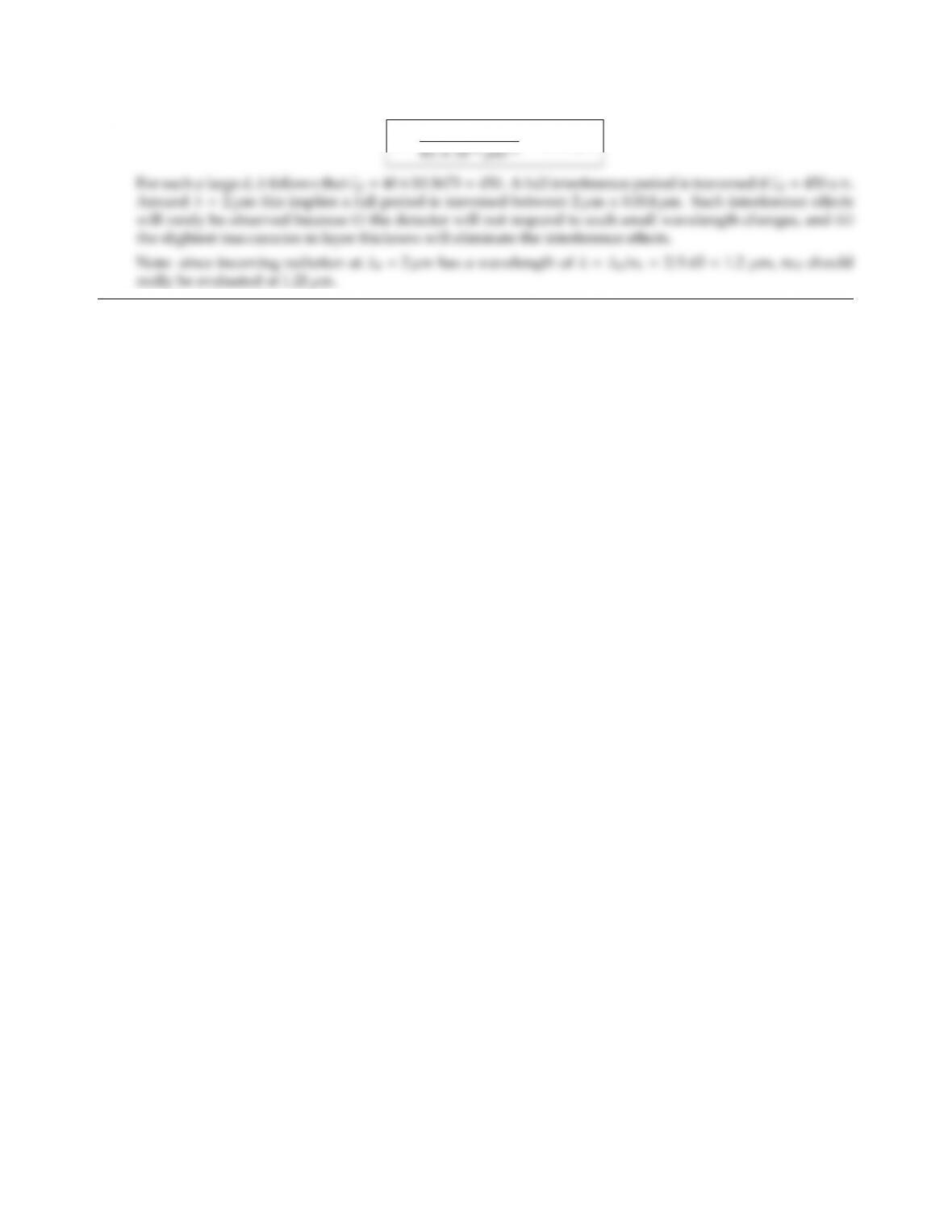# 978-0123869449 Chapter 2

Document Type
Homework Help
Book Title
Authors
Michael F. Modest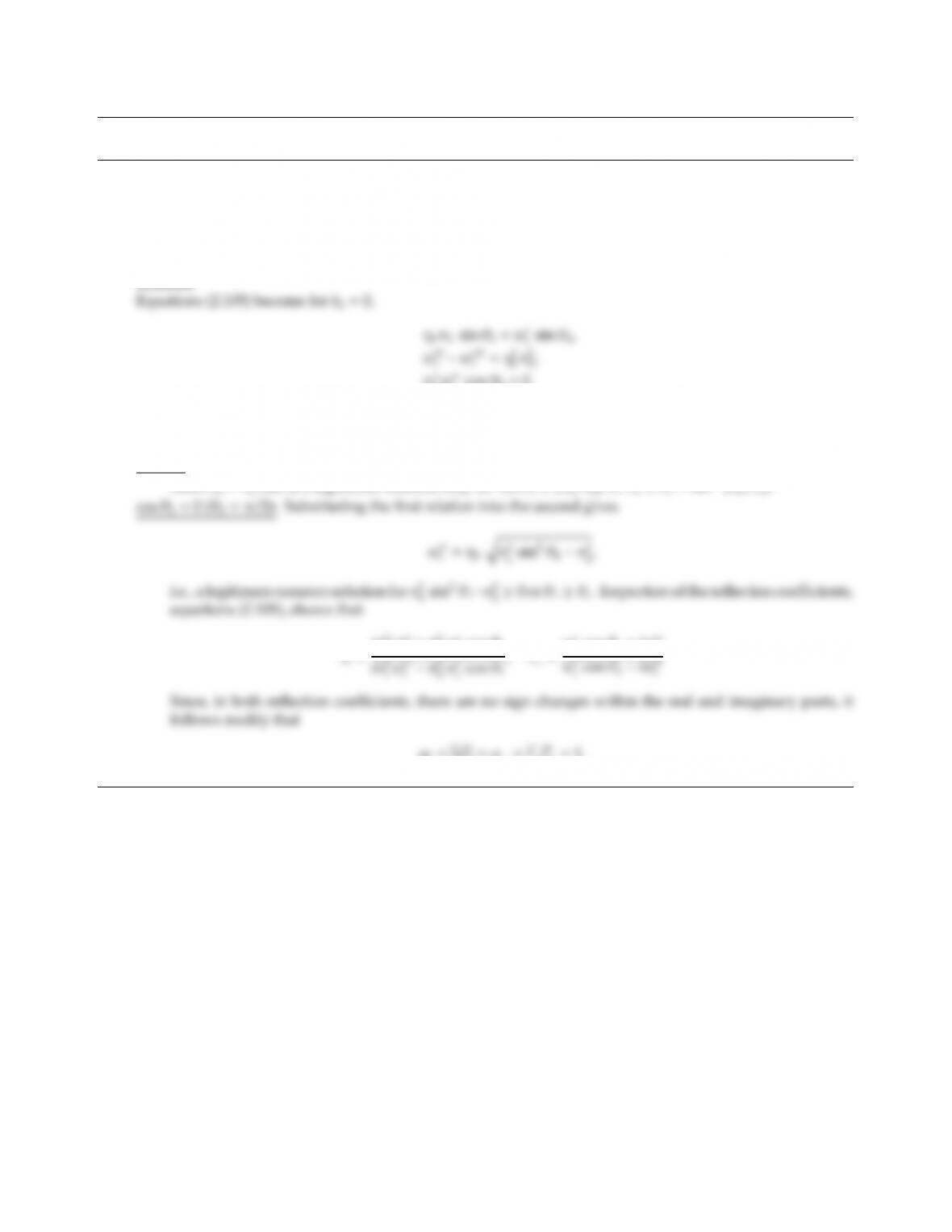CHAPTER 2
2.1 Show that for an electromagnetic wave traveling through a dielectric (m1=n1), impinging on the interface
with another, optically less dense dielectric (n2<n1), light of any polarization is totally reﬂected for incidence
angles larger than θc=sin1(n2/n1).
Hint: Use equations (2.105) with k2=0.
Solution
w
tw′′
tcos θ2=0.
The last of these relations dictates that either w′′
t=0 or cos θ2=0 (w
t=0 is not possible since—from the first
relation—this would imply η0n1sin θ1=0 which is known not to be true).
w′′
t=0:Substituting this into the second relation leads to w
t=η0n2, and the first leads to n1sin θ1=n2sin θ2.
Since n1>n2this is a legitimate solution only for sin θ1(n2/n1), or θ1θc=sin1(n2/n1).
e
rk=in2
1w′′
t+n2
2w
icos θ1
,e
r=w
icos θ1+iw′′
t
ρk=e
rke
r
k=ρ=e
re
r
=1.
19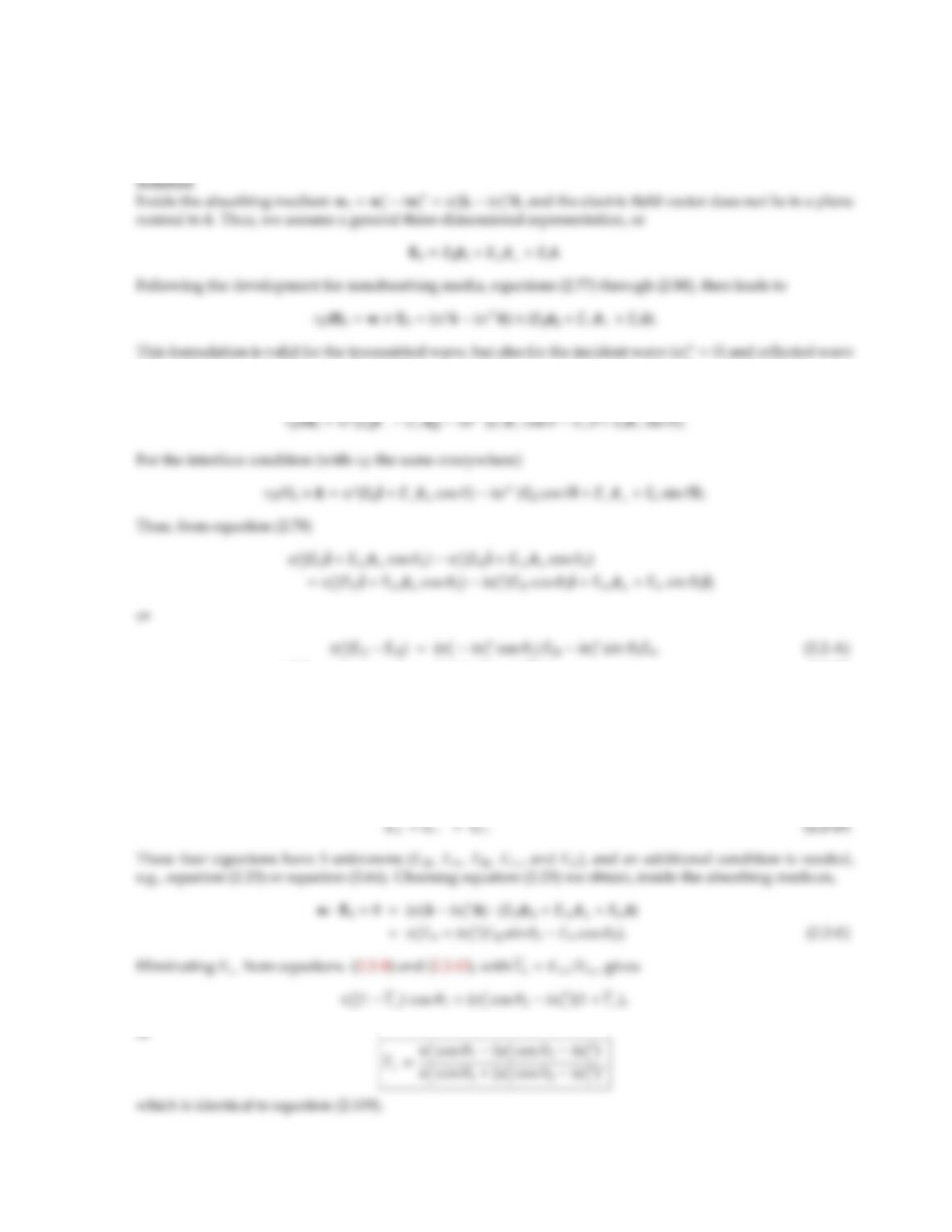2.2 Derive equations (2.109) using the same approach as in the development of equations (2.89) through (2.92).
Hint: Remember that within the absorbing medium, w=wiw′′ =wˆ
siw′′ ˆ
n; this implies that E0is not a
vector normal to ˆ
s. It is best to assume E0=Ekˆ
ek+Eˆ
e+Esˆ
s.
i=0) and reﬂected wave
(w′′
r=0,w
r=w
i). The contribution from Esvanishes for incident and reﬂected wave. Using the same
vector relations as given for the nonabsorbing media interface, one obtains
w
i(EiEr) cos θ1=(w
tcos θ2iw′′
t)Et(2.2-B)
Similarly, from equation (2.77),
E0×ˆn =(Ekˆek+Eˆe+Esˆs)×ˆn =Ekˆecos θ+Eˆ
tEsˆesin θ
and
(Eik+Erk) cos θ1=Etkcos θ2+Ets sin θ2(2.2-C)
or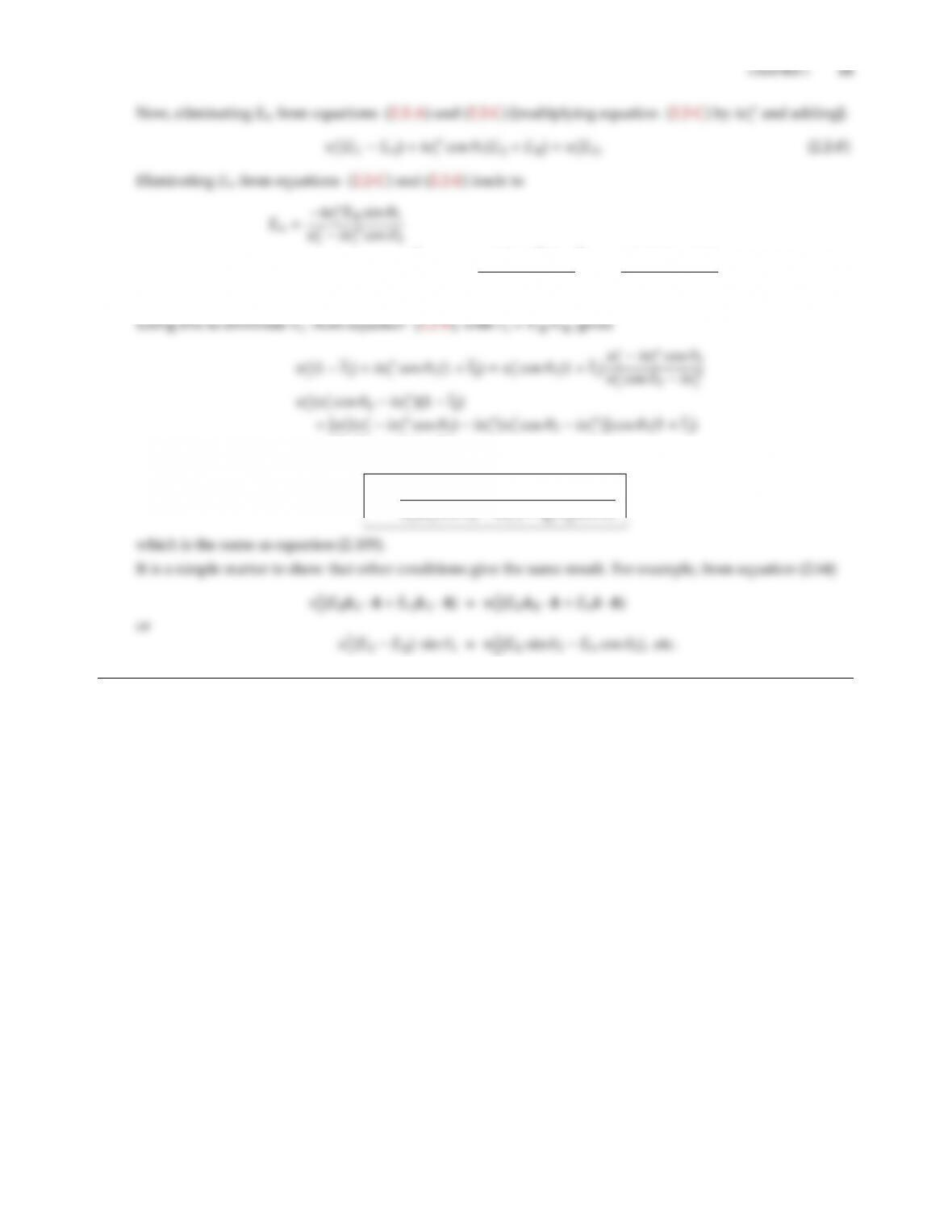(Eik+Erk) cos θ1=Etkcos θ2iw′′
tsin2θ2
w
tiw′′
tcos θ2=Etk
w
tcos θ2iw′′
t
w
tiw′′
tcos θ2
.
=η2
0m2
2cos θ1(1 +e
rk),
e
rk=w
i(w
tcos θ2iw′′
t)η2
0m2
2cos θ1
w
,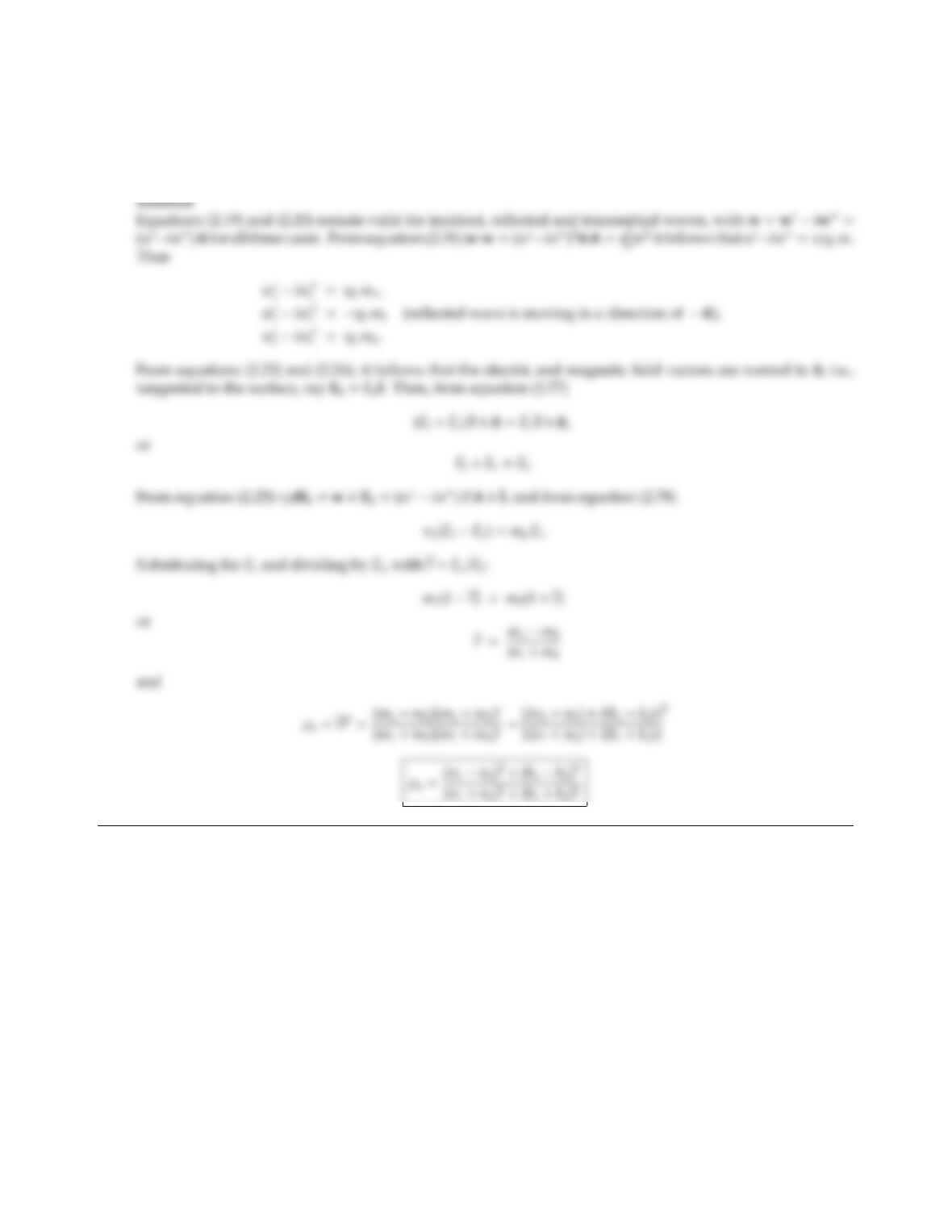2.3 Find the normal spectral reﬂectivity at the interface between two absorbing media. [Hint: Use an approach
similar to the one that led to equations (2.89) and (2.90), keeping in mind that all wave vectors will be complex,
but that the wave will be homogeneous in both media, i.e., all components of the wave vectors are colinear
with the surface normal].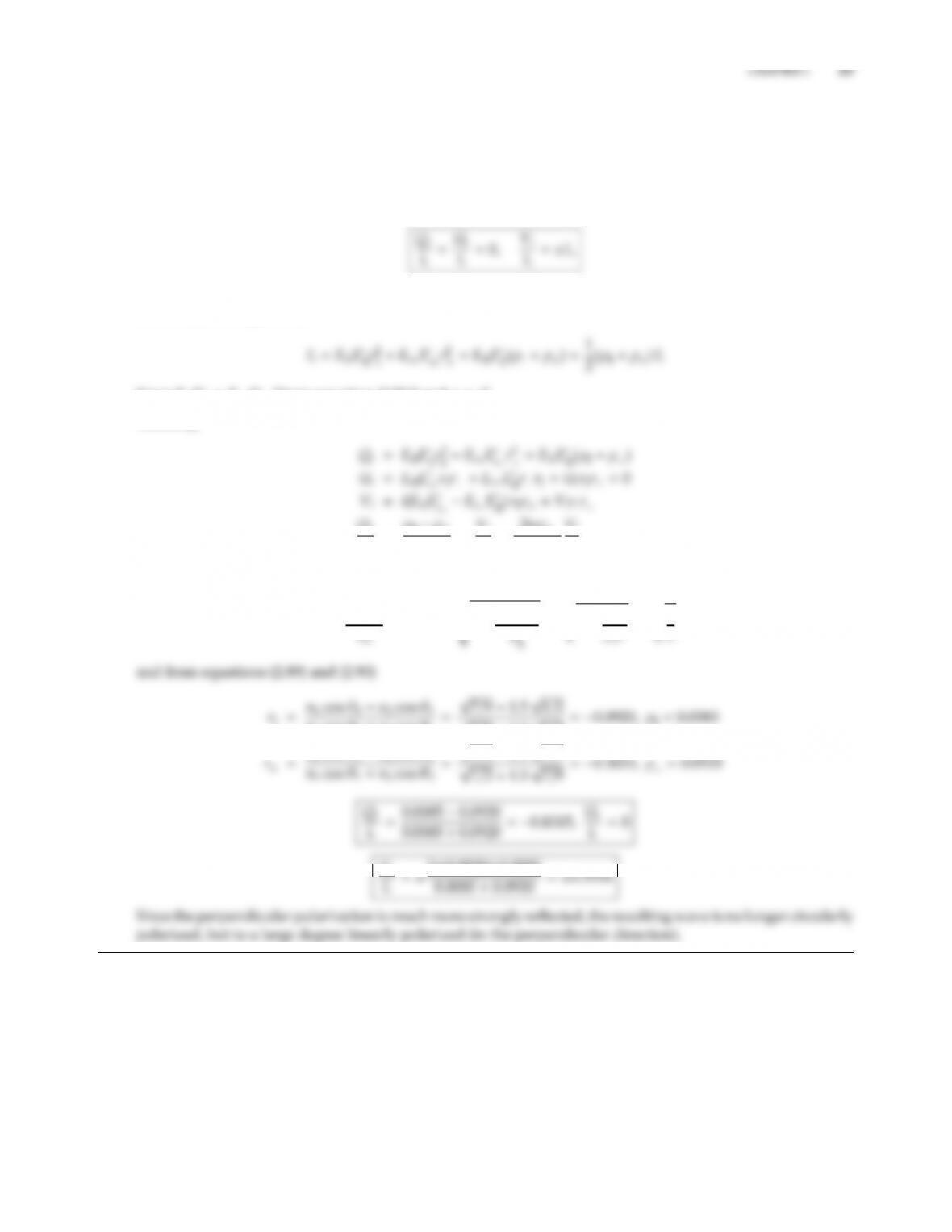2.4 A circularly polarized wave in air is incident upon a smooth dielectric surface (n=1.5) with a direction of
45onormal. What are the normalized Stokes’ parameters before and after the reﬂection, and what are the
degrees of polarization?
Solution
From the definition of Stokes’ parameters the incident wave has degrees of polarization
the sign giving the handedness of the circular polarization. With Erk=Eikrkand Er=Eir, from equa-
tions (2.50) through (2.53):
Since EikE
ik=EiE
i[from equation (2.51)] and ρ=r2.
Similarly,
Qr
Ir
=ρkρ
ρk+ρ
,Vr
Ir
=2rkr
ρk+ρ
Vi
Ii
.
From Snell’s law
sin θ2=sin θ1
; cos θ2=s1sin2θ1
=r10.5
n1cos θ2+n2cos θ1
Vr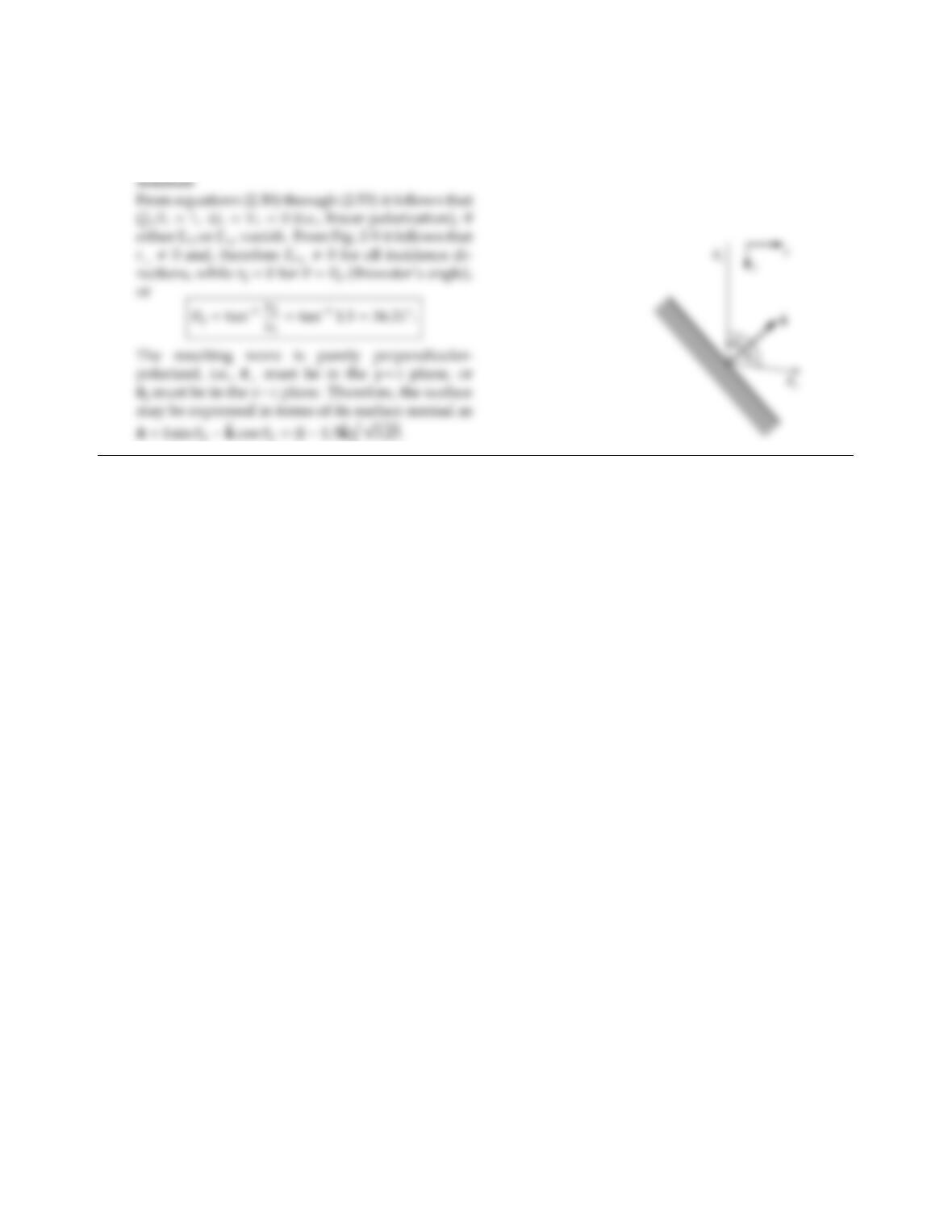2.5 A circularly polarized wave in air traveling along the z-axis is incident upon a dielectric surface (n=1.5).
How must the dielectric-air interface be oriented so that the reﬂected wave is a linearly polarized wave in the
y-z-plane?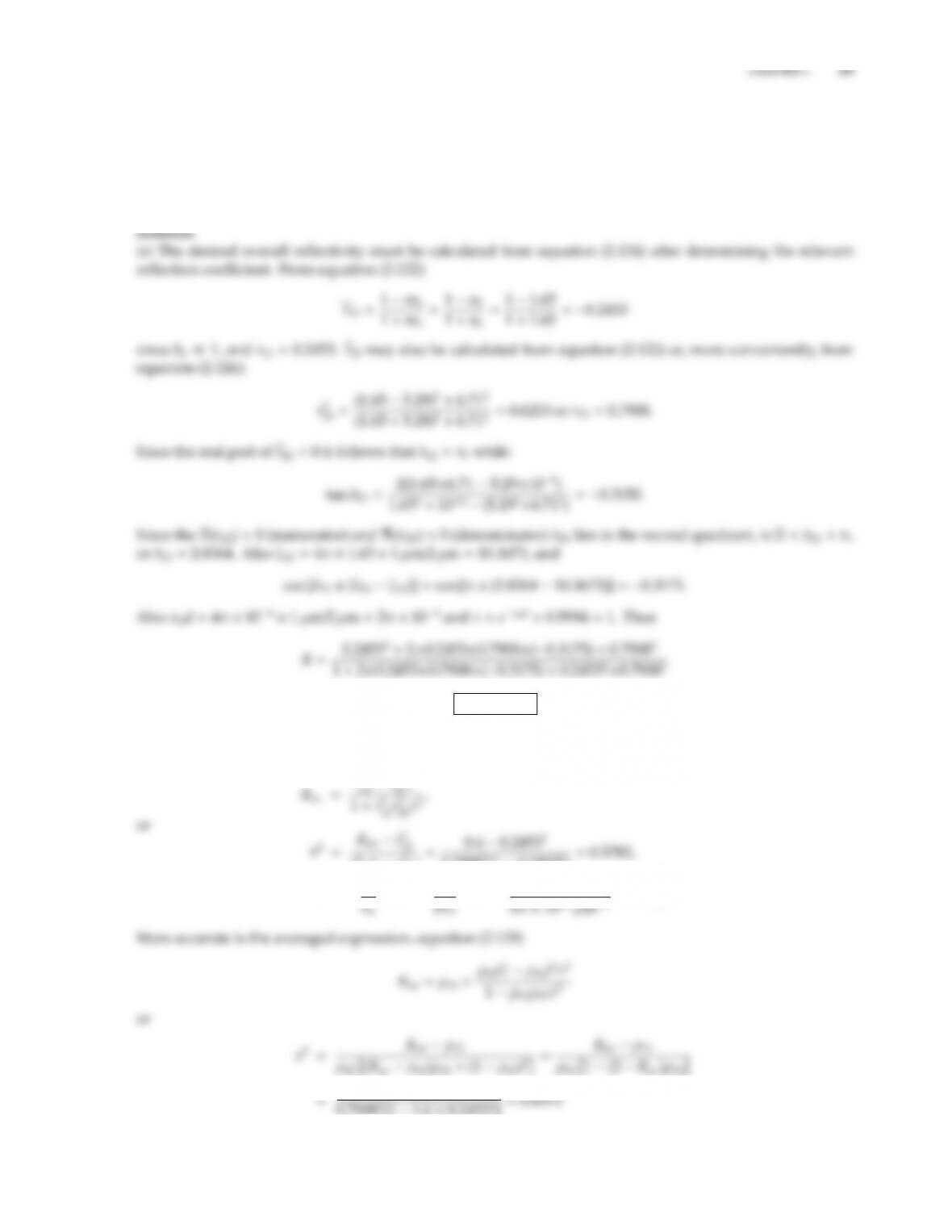2.6 A polished platinum surface is coated with a 1 µm thick layer of MgO.
(a) Determine the material’s reﬂectivity in the vicinity of λ=2µm (for platinum at 2 µmmPt =5.29 6.71 i,
for MgO mMgO =1.65 0.0001 i).
(b) Estimate the thickness of MgO required to reduce the average reﬂectivity in the vicinity of 2 µm to 0.4.
What happens to the interference eects for this case?
R=0.6149.
(b) The cos in the numerator ﬂuctuates between 1<cos <+1. The average value for Ris obtained by
dropping the cos-term. Then
r2
23(1 r2
12)=0.40.24532
0.79082(1 0.24532)=0.5782,
d=1
ln τ=1
ln τ2=ln 0.5782
0.79082[1 1.6×0.24532]=0.6013